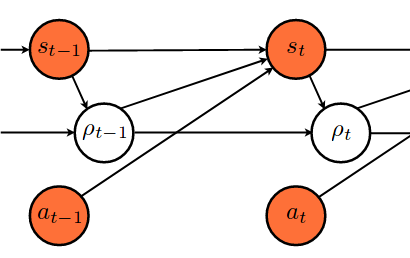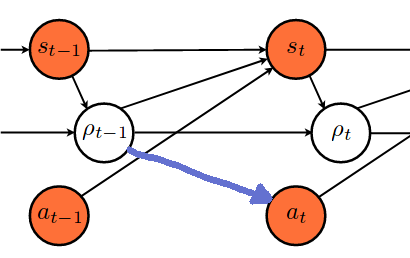# Implementing a custom graphical model

Hi there,

I am very new to pyro and want to implement a graphical model / HMM / Baysesian network that looks like thisand if possible later one which has an additional connectionwhere the orange color marks observed variables.

So far, I read the HMM tutorial, but the closest model there is the Dynamic Bayesian Network (model_4), which has 2 hidden states and 1 observation. In the case I want to implement, there is 1 discrete hidden state and 2 continious observable states.

Do I understand it correctly, that the number in `to_event` expresses causal ordering?
Can/Should I use parallel enumeration as discribed in this tutorial?

I am thankful for a point (e.g. code snippet) to start from.

Hi @famura, That looks like an interesting model, and very suitable for Pyro.

Do I understand it correctly, that the number in `to_event` expresses causal ordering?

No, rather `.to_event()` is a way to change how Pyro interpret’s the shape of a distribution. Pyro distributions (like PyTorch and Tensorflow distributions) split shape into batch_shape + event_shape, where things are iid over batch_shape but may have dependencies among event_shape. `.to_event()` tells pyro to treat sum number of batch dims as event dims even though they are iid. This is useful e.g. for producing a diagonal normal distribution.

Can/Should I use parallel enumeration …?

Yes you can use parallel enumeration. Your model should look something like

``````@config_enumerate
def model(s, a):
assert len(s) == len(a)
for t in pyro.markov(range(len(s))):
if t == 0:  # initial step
pyro.sample("s_0", dist.Normal(s_init_fn(), 1), obs=s[t])
pyro.sample("a_0", dist.Normal(a_init_fn(), 1), obs=a[t])
rho = pyro.sample("rho_0",
dist.Categorical(rho_init_fn(s[t])))
else:
pyro.sample("s_{}".format(t),
dist.Normal(s_step_fn(s[t-1], rho[t-1], a[t-1]), 1), obs=s[t])
pyro.sample("a_{}".format(t),
dist.Normal(a_step_fn(rho[t-1]), 1), obs=a[t])
rho = pyro.sample("rho_{}".format(t),
dist.Categorical(rho_step_fn(s[t], a[t])))
``````

You should be able to train using TraceEnum_ELBO and should be able to sample `rho` from the posterior or MAP estimate `rho` using infer_discrete.

1 Like

Hi @fritzo, that you very much for your detailed answer. I will give it a try!

One final thing: from the fact that you did not mention the guide I infer that the auto guide will do just fine.

Hi @famura, because all of your latent variables are discrete and you are using enumeration, you can use an empty guide:

``````def guide(s, a):
pass
``````

In this case Stochastic Variational Inference becomes trivial, and you’ll really be doing deterministic first-order Baum-Welch learning. If you later want to make this a hierarchical model, you could include latent global continuous variables and use an `AutoGuide` to learn those variables.